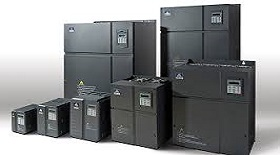## The structure of VFD

1. Rectifier. A large number of diodes are used as converters, which convert power to dc power. Two groups of transistor converters can also be used to form a reversible converter, which can be regenerated and operated due to its reversible power direction.

2. Flat wave loop. In the dc voltage after rectifier rectification, there is pulsating voltage with 6 times frequency of power supply. In addition, the pulsating current produced by inverter also makes dc voltage change. To suppress voltage fluctuation, inductance and capacitance are used to absorb pulsating voltage (current). If the power supply and main circuit components have a surplus, inductance can be omitted by using a simple flat wave loop when device capacity is small.

3. Inverter. In contrast to the rectifier, the inverter changes the dc power into the required frequency of ac power, and the 6 switching devices can be switched on and off to get 3 phase ac output with the determined time. The switching time and voltage waveform are shown by taking the voltage type PWM inverter as an example.

Control circuit for asynchronous motor power supply (voltage, frequency adjustable) of main circuit provides the control signal circuit. It has "operation circuit" of frequency and voltage, "the voltage, current detection circuit" of main circuit, "speed detection circuit" of motor, "drive circuit" of the operation circuit of the control signal amplification, and "protection circuit" of inverter and motor.

(1) Operational circuit: the external speed, torque and other instructions are compared with the current and voltage signals of the detection circuit to determine the output voltage and frequency of the inverter.

(2) Voltage and current detection circuit: the voltage and current are isolated from the main circuit potential.

(3) Driving circuit: the circuit that drives the main circuit device. It is isolated from the control circuit to make the main circuit components on and off.

(4) Speed detection circuit: the signal of the speed detector (tg, PLG, etc.) installed on the shaft of the asynchronous motor is the speed signal, which is sent to the operation loop, and the motor can operate according to the command and operation.

(5) Protection circuit: detecting the voltage and current of the main circuit and so on, in case of overload or overvoltage, etc., in order to prevent damage of inverter and asynchronous motor.